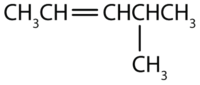# 7.5: Alkenes and Alkynes

$$\newcommand{\vecs}{\overset { \rightharpoonup} {\mathbf{#1}} }$$ $$\newcommand{\vecd}{\overset{-\!-\!\rightharpoonup}{\vphantom{a}\smash {#1}}}$$$$\newcommand{\id}{\mathrm{id}}$$ $$\newcommand{\Span}{\mathrm{span}}$$ $$\newcommand{\kernel}{\mathrm{null}\,}$$ $$\newcommand{\range}{\mathrm{range}\,}$$ $$\newcommand{\RealPart}{\mathrm{Re}}$$ $$\newcommand{\ImaginaryPart}{\mathrm{Im}}$$ $$\newcommand{\Argument}{\mathrm{Arg}}$$ $$\newcommand{\norm}{\| #1 \|}$$ $$\newcommand{\inner}{\langle #1, #2 \rangle}$$ $$\newcommand{\Span}{\mathrm{span}}$$ $$\newcommand{\id}{\mathrm{id}}$$ $$\newcommand{\Span}{\mathrm{span}}$$ $$\newcommand{\kernel}{\mathrm{null}\,}$$ $$\newcommand{\range}{\mathrm{range}\,}$$ $$\newcommand{\RealPart}{\mathrm{Re}}$$ $$\newcommand{\ImaginaryPart}{\mathrm{Im}}$$ $$\newcommand{\Argument}{\mathrm{Arg}}$$ $$\newcommand{\norm}{\| #1 \|}$$ $$\newcommand{\inner}{\langle #1, #2 \rangle}$$ $$\newcommand{\Span}{\mathrm{span}}$$

##### Learning Objectives
• To name alkenes given formulas and write formulas for alkenes given names.

As noted before, alkenes are hydrocarbons with carbon-to-carbon double bonds (R2C=CR2) and alkynes are hydrocarbons with carbon-to-carbon triple bonds (R–C≡C–R). Collectively, they are called unsaturated hydrocarbons because they have fewer hydrogen atoms than does an alkane with the same number of carbon atoms, as is indicated in the following general formulas:Some representative alkenes—their names, structures, and physical properties—are given in Table $$\PageIndex{1}$$.

Table $$\PageIndex{1}$$: Physical Properties of Some Selected Alkenes
IUPAC Name Molecular Formula Condensed Structural Formula Melting Point (°C) Boiling Point (°C)
ethene C2H4 CH2=CH2 –169 –104
propene C3H6 CH2=CHCH3 –185 –47
1-butene C4H8 CH2=CHCH2CH3 –185 –6
1-pentene C5H10 CH2=CH(CH2)2CH3 –138 30
1-hexene C6H12 CH2=CH(CH2)3CH3 –140 63
1-heptene C7H14 CH2=CH(CH2)4CH3 –119 94
1-octene C8H16 CH2=CH(CH2)5CH3 –102 121

We used only condensed structural formulas in Table $$\PageIndex{1}$$. Thus, CH2=CH2 stands forThe double bond is shared by the two carbons and does not involve the hydrogen atoms, although the condensed formula does not make this point obvious. Note that the molecular formula for ethene is C2H4, whereas that for ethane is C2H6.

The first two alkenes in Table $$\PageIndex{1}$$, ethene and propene, are most often called by their common names—ethylene and propylene, respectively (Figure $$\PageIndex{1}$$). Ethylene is a major commercial chemical. The US chemical industry produces about 25 billion kilograms of ethylene annually, more than any other synthetic organic chemical. More than half of this ethylene goes into the manufacture of polyethylene, one of the most familiar plastics. Propylene is also an important industrial chemical. It is converted to plastics, isopropyl alcohol, and a variety of other products.Figure $$\PageIndex{1}$$: Ethene and Propene. The ball-and-spring models of ethene/ethylene (a) and propene/propylene (b) show their respective shapes, especially bond angles.

Although there is only one alkene with the formula C2H4 (ethene) and only one with the formula C3H6 (propene), there are several alkenes with the formula C4H8.

Here are some basic rules for naming alkenes from the International Union of Pure and Applied Chemistry (IUPAC):

1. The longest chain of carbon atoms containing the double bond is considered the parent chain. It is named using the same stem as the alkane having the same number of carbon atoms but ends in -ene to identify it as an alkene. Thus the compound CH2=CHCH3 is propene.
2. If there are four or more carbon atoms in a chain, we must indicate the position of the double bond. The carbons atoms are numbered so that the first of the two that are doubly bonded is given the lower of the two possible numbers.The compound CH3CH=CHCH2CH3, for example, has the double bond between the second and third carbon atoms. Its name is 2-pentene (not 3-pentene).
3. Substituent groups are named as with alkanes, and their position is indicated by a number. Thus, the structure below is 5-methyl-2-hexene. Note that the numbering of the parent chain is always done in such a way as to give the double bond the lowest number, even if that causes a substituent to have a higher number. The double bond always has priority in numbering.##### Example $$\PageIndex{1}$$

Name each compound.

1.1.#### Solution

1. The longest chain containing the double bond has five carbon atoms, so the compound is a pentene (rule 1). To give the first carbon atom of the double bond the lowest number (rule 2), we number from the left, so the compound is a 2-pentene. There is a methyl group on the fourth carbon atom (rule 3), so the compound’s name is 4-methyl-2-pentene.
2. The longest chain containing the double bond has five carbon atoms, so the parent compound is a pentene (rule 1). To give the first carbon atom of the double bond the lowest number (rule 2), we number from the left, so the compound is a 2-pentene. There is a methyl group on the third carbon atom (rule 3), so the compound’s name is 3-methyl-2-pentene.
##### Exercise $$\PageIndex{1}$$

Name each compound.

1. CH3CH2CH2CH2CH2CH=CHCH3
•Just as there are cycloalkanes, there are cycloalkenes. These compounds are named like alkenes, but with the prefix cyclo- attached to the beginning of the parent alkene name.

##### Example $$\PageIndex{2}$$

Draw the structure for each compound.

1. 3-methyl-2-pentene
2. cyclohexene
###### Solution
1. First write the parent chain of five carbon atoms: C–C–C–C–C. Then add the double bond between the second and third carbon atoms:Now place the methyl group on the third carbon atom and add enough hydrogen atoms to give each carbon atom a total of four bonds.• First, consider what each of the three parts of the name means. Cyclo means a ring compound, hex means 6 carbon atoms, and -ene means a double bond.##### Exercise $$\PageIndex{2}$$

Draw the structure for each compound.

1. 2-ethyl-1-hexene
2. cyclopentene

## Key Takeaway

• Alkenes are hydrocarbons with a carbon-to-carbon double bond.

7.5: Alkenes and Alkynes is shared under a not declared license and was authored, remixed, and/or curated by LibreTexts.